American Journal of Theoretical and Applied Statistics
Volume 5, Issue 4, July 2016, Pages: 180-185

Feed Forward Neural Network Versus Kernel Regression a Case of Body Mass Index and Body Dimensions

Nzinga Christine Mutono1, Gichuhi Anthony Waititu2, Wanjoya Anthony Kiberia2

1Applied Statistics, Department of Statistics and Actuarial Science, Jomo Kenyatta University of Agriculture and Technology, Nairobi, Kenya

2Statistics, Department of Statistics and Actuarial Science, Jomo Kenyatta University of Agriculture and Technology, Nairobi, Kenya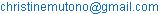(N. C. Mutono)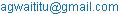(G. A. Waititu)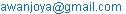(W. A. Kiberia)

Nzinga Christine Mutono, Gichuhi Anthony Waititu, Wanjoya Anthony Kiberia. Feed Forward Neural Network Versus Kernel Regression a Case of Body Mass Index and Body Dimensions. American Journal of Theoretical and Applied Statistics. Vol. 5, No. 4, 2016, pp. 180-185. doi: 10.11648/j.ajtas.20160504.13

Received: May 5, 2016; Accepted: May 18, 2016; Published: June 7, 2016

Abstract: Body mass index is a measure of body fitness and is considered very important in screening body categories that may lead to health problems. Understanding risk factors of obesity provide more insight and nature of policies that can be put up to fight obesity. However, uncertainty regarding most appropriate means by which to define excess body weight remains. It is important to develop models that best calculate Body Mass Index to help reduce the chances of obesity. The objective of this research ismodeling Body Mass Index using Feed Forward Neural Network and Kernel regression. Modeling will be first done using height and weight alone, later 21 body dimensions will be added. The analysis was based on body dimensions data provided by San Jose State University and the U.S. Naval Postgraduate School in Monterey, California. To determine the best model, Adjusted R2 and Mean Square Error (MSE) were used. From the results of the study, Kernel regression was better in modeling Body Mass Index than Feed Forward Neural Network.

Keywords: Feed Forward Neural Network, Body Mass Index (BMI), Artificial Neural Network (ANN), Kernel Regression

1. Introduction

1.1. Background of the Study

Better health is central to human happiness and well-being. It also makes an important contribution to economic progress, as healthy populations live longer, are more productive, and save more. Body Mass Index (BMI) is used as a measure of persons' fitness and is therefore considered a very important measure by health professionals. Using BMI one maybe categorized as underweight while the other overweight due to percentage of body fat. Understanding which factors influence individual body weight and how exactly excess body fat is contributing to increase risk for disease may help to reduce the increased prevalence of several common disorders associated with obesity, thereby lessening the burden placed on health care systems. Men are said to move up the rank of BMI as compared to women. BMI is termed as an indirect measure of body fat and it indicates weight-for-height without considering differences in body composition and the contribution of body fat to overall body weight. One of the most appealing features of nonparametric estimation techniques is that, by allowing the data to model the relationships among variables, they are robust to functional form specification and therefore have the ability to detect structure which sometimes remains undetected by traditional parametric estimation techniques. A feed forward network is an artificial neural network where connections between the units do not form a directed cycle. The feed forward neural network was the first and simplest type of artificial neural network devised. Information moves only in one direction, forward from input nodes through hidden nodes (if any) and to output nodes. There are no cycles or loops in the network.

1.2. Statement of the Problem

Body Mass Index is used for screening weight categories as either underweight, normal or overweight and is measured in kg/m2. The obesity epidemic in adults is an enormous societal problem with far reaching consequences. Overweight and obese adults also have higher rates of high blood pressure; abnormal insulin levels among other health problems and is associated with decreased survival. Body Mass Index traits are influenced by both genetic and non genetic factors and provide a simple measure of a persons’ thickness. Body Mass Index has always been calculated using height and weight alone without considering the effect of other body dimensions like it ignores waist size, which is a clear indicator of obesity level. Incorrect BMI categorization may prevent some people from receiving necessary weight loss help and may mislabel others as overweight when they have a healthy percentage of body fat. There is therefore a need to develop better models that give more accurate Body Mass Index values. The developed models can therefore be applied when calculating BMI to reduce the risk of obesity and this will help in improving health in any population. Kvaavik et al. (2003) showed that women are more likely than men to move down in BMI rank, while men tend to move up in BMI rank.

1.3. Justification of the Study

World Health Organization (WHO) states that for adults, the healthy range for BMI is between (18.5 and 24.9), less than 18.5 is underweight while greater than 24.9 is overweight. BMI provides simple measure of a persons' thickness allowing health professionals to discuss weight problems more objectively with their patterns. This project came up with a more accurate statistical model that predicts Body Mass Index using other body dimensions other than height and weight alone. The study develops models using Feed Forward Neural Network and kernel regression techniques. These techniques are chosen because the predictor does not take a predetermined form but is constructed according to information derived from the data. The two models are then compared and best developed model can be used to reduce chances of obesity.

1.4. Objectives

1.4.1. General Objective

The drive of this study is to compare the performance of feed forward neural network and kernel regression.

1.4.2. Specific Objective

1. To model Body Mass Index data using feed forward neural network.

2. To model Body Mass Index data using kernel regression function.

3. To compare the performance of two modeling techniques.

4. To investigate effect of gender on Body Mass Index.

2. Review of the Previous Studies

3. Research Methodology

3.1. Introduction

In this section, we discuss Feed forward neural networks used. We then discuss kernel regression and its procedure. Lastly, the model performance measures.

3.2. Feed Forward Neural Networks

Feed forward neural networks is an artificial neural network which represent a function of explanatory variables which is composed of simple building blocks and which will be used to provide an approximation of conditional expectations. Connections between units do not form a directed cycle. Artificial neural network is a parallel connection of a set of nodes called neurodes (weights).

Input at hidden layer nodes are connected by weights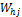for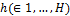and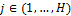where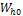is the bias of the ithhidden node. The hidden and output layers are connected by weights forand. Considering an input vector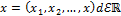, and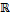is the real line, the input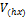to the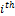hidden node is the value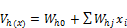(1)

The output becomes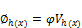(2)

Training a Neural Network

The Sum of squared error (SSE) is used to train faced forward networks. In this method the weights are adjusted in such a way that the SSE between the targets y and the goal of output Z is minimized.

The SSE is defined as: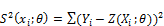(3)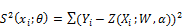(4)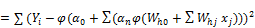(5)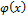is activation function which is used to transform the activation level of a unit (neuron) into an output signal.

3.3. Kernel Regression

One of the most popular methods for nonparametric kernel regression was proposed by Nadaraya  Watson  and is known as the "Nadaraya–Watson" estimator though it is also known as the "local constant" estimator for reasons best described when we introduce the "local polynomial" estimator. Kernel simply means a weighted function and the primary role of the kernel is to impart smoothness and differentiability on the resulting estimator. The appeal on non-parametric methods lies in the ability to reveal structure in data that might be missed by classical parametric method. Kernel methods have the potential to recapture the efficiency losses associated with non-parametric frequency approaches as they do not rely on sample splitting rather they smooth the categorical variables in appropriate manner Li and Racine . Kernel density estimation approach overcomes the discreteness of histogram approach by centering a smooth kernel function at each data point then summing to get a density estimate. The common kernel functions include uniform, triangle, Epanochnkoz, biweight, tricube, Gaussian and cosine. Kernel density estimate approach has a problem in varying data density; regions of high data density could have small h while sparse data need large h. To overcome this problem we allow bandwidth to vary Nadaraya  Watson ) proposed to estimate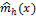as a locally weighted average using kernel as a weighting function. The Nadaraya Watson estimator is given by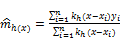(6)

Wherek is the kernel and h is the bandwidth.

Bandwidth Selection

The key to sound nonparametric estimation lies in selecting an appropriate bandwidth for the problem at hand. Least squares cross validation is a data driven bandwidth selection method. Typically bandwidth is chosen by minimizing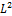risk, Meanintegrated square errors (MISE).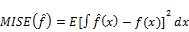(7)

3.4. Model Performance Measures

To estimate the best model among the two, Adjusted R2 and mean squared error was used.

3.4.1. Non-parametric R2

The model that has the highest value ofR2 is the best model. Let yi denote the observed value and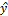i denote the fitted value for observation i. Let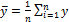i for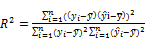(8)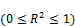The use of an adjusted R2 is an attempt to take account of the phenomenon of the R2 automatically increasing when extra explanatory variables are added to the model. Adjusted R2 is defined as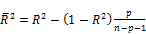(9)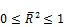Wherep is the total number of explanatory variables in the model (not including the constant term), and n is the sample size. The model with the highest value of adjusted R2 is the best model.

3.4.3. Mean Square Error (MSE)

A common and convenient measure of estimation precision is the mean squared error and it measures the average of the squares of the error that is the difference between the estimator and what is estimated.

It is defined by the following equation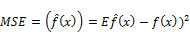(10)

A model with least MSE is the better model fit.

3.5. Wilcoxon Rank Sum Test

Wilcoxon rank sum test compares the medians from two populations and works when the Y variable is continuous, discrete-ordinal or discrete-count, and the X variable is discrete with two attributes. In this test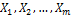are identically independent distribution function ofare identically independent distribution function of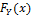. Let M1 be the median for distribution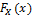and M2 median for distribution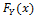. Then M1-M2=0 will be denoted by dm.

To test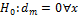Against a suitable alternative hypothesis.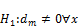This test assumes there is no difference between the medians.

3.6. Description of the Data

In this study, there was consideration of nine skeletal measurements. These included biacromial, biiliac, bitrochanteric and chest diameters. These measurements were done using anthropometer. To get the measurements of the other four skeletal measurements which included elbow, wrist, knee and ankle there was use of a smaller anthropometer. At this age it was noted that measurements like height already attained maximum size. Twelve girth measurements which included shoulder, chest, waist, hip, bicep, thigh, calf, forearm, navel, wrist, ankle and knee were included in the study. These measurements however are not fixed but vary over time except only the wrist, knee and ankle which are most likely to remain constant over time. The other measurements included in the study was height and weight and this was done for individuals in their twenties and a few individuals in their thirties. The total number of explanatory variables under consideration was therefore 23.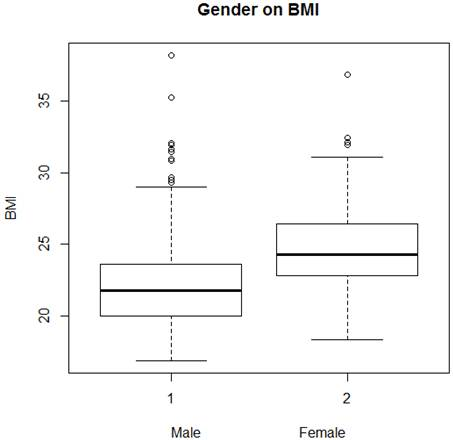Figure 1. Box plot of gender on BMI.

Figure 1 shows a box plot of gender on BMI, male have more extreme values (shown as circles separated from the box) or large departures from symmetry while female have fewer. Box plots are used to show overall patterns of response for a group. They provide a useful way to visualize the range and other characteristics of responses for a large group. The median is indicated by the horizontal line that runs across the center of the box. In the box plot above the median for male is approximately 22 while for female is approximately 24 and therefore the BMI of female is higher than the BMI for male.

4. Results and Discussion

4.1. Introduction

Feed forward neural network utilizes the nnet package while Kernel regression estimate was done using the add-on package "np" for nonparametric regression and nonparametric specification tests. This chapter describes how Feed Forward neural network and Kernel regression were used to model BMI. The chapter also describes how the modeling results from both models were compared.

4.2. Selecting Best Feed Forward Neural Network Model for BMI

Multiple values of MSE were calculated in order to determine the optimal number of hidden nodesfor 2 explanatory variables and was found to be 2 hidden nodes with MSE of 3.2199, the optimal hidden nodes is given by the least MSE. These hidden nodes gave Adjusted R2 of 0.98518.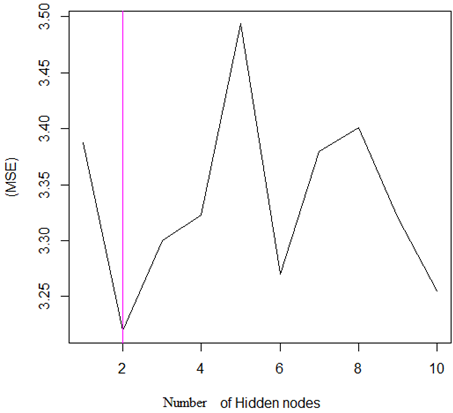Figure 2. MSE against Number of hidden nodes.

Multiple values of MSE were calculated in order to determine the optimal number of hidden nodes for 23 explanatory variablesand was found to be 2 hidden nodes with MSE of 2.85234, the optimal hidden nodes is given by the least MSE. These hidden nodes gave Adjusted R2 of 0.98523.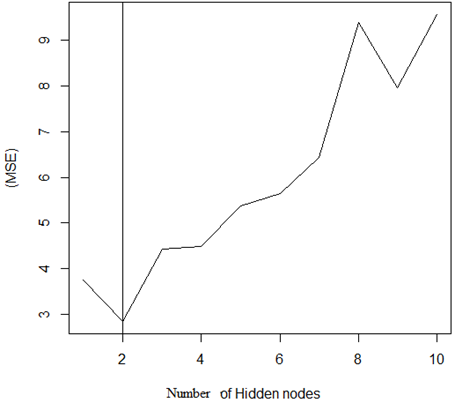Figure 3. MSE against Number of hidden nodes.

From the study the model with 23 explanatory variables was a better model.

4.3. Selecting Best Kernel Regression Estimate for BMI

Table 1. Model summary for kernel regression with 2 explanatory variables.

 Regression Data 507 training points, in 2 variable (s) Bandwidth Type Fixed Formula BMI~weight+height weight Bandwidth:1.027492 height Bandwidth:0.01021961 Kernel Regression Estimator Local-Constant Residual standard error 0.108701 Continuous Kernel Type Second-Order Gaussian

For Kernel regression estimate with 2 explanatory variables, Adjusted R2 of 0.998967 and MSE of 0.01182.

Table 2. Model summary for kernel regression with 23explanatory variables.

 Regression Data 507 training points, in 23 variable (s) Bandwidth Type Fixed Formula BMI~weight+height+21variables Kernel Regression Estimator Local-Constant Residual standard error 0.095218 Continuous Kernel Type Second-Order Gaussian

For Kernel regression estimate with 23 explanatory variables Adjusted R2 of 0.99908 and MSE of 0.00906. The study thus conclude that the model with 23 explanatory is the better fit.

4.4. Performance Statistics of Feed Forward Neural Networks and Kernel Regression Models

Table 3. Performance Statistics.

 No. of explanatory variables Adjusted R2 MSE Feed forward neural network 2 0.98518 3.2199 23 0.98523 2.85234 Kernel Regression 2 0.99897 0.01182 23 0.99908 0.00906

From the table 3, using adjusted R2 and MSE we conclude that kernel regression model performs better than Feed forward neural network model in calculating BMI.

4.5. Testing the Effect of Gender on BMI

Using wilcoxon signed Rank test, the statistic W is 16100 and the P-value<2.26e-16. The p-value is less than 0.5% significance level and therefore we reject the null hypothesis and conclude that the median of male and female are significant. The study thus concludes that gender has an impact on BMI.

5. Conclusion and Recommendations

5.1. Conclusion

This chapter presents summary of key findings and conclusion drawn from the study. The main objective of this study was to compare performance of Feed forward neural network and kernel regression models in calculating BMI. Kernel regression model and Feed forward neural network model can effectively calculate BMI. However kernel regression is considered as the best model in this study. This is because of the ability of kernel regression to have data-driven methods of bandwidth selection. Nonparametric kernel smoothing methods have experienced tremendous growth in recent years, and are being adopted by applied researchers across a range of disciplines. Kernel approaches offer a set of potentially useful methods to those who must confront the vexing issue of parametric model misspecification. The appeal of nonparametric methods lies in their ability to reveal structure in data that might be missed by classical parametric methods.

5.2. Recommendations

We recommend that in calculation of Body Mass Index it is important to consider the effect of other body dimensions other than weight and height alone. We also recommend future research usinglocally weighted regression (LOWESS) and smoothing splines.

References

1. Cizek, P and W Hardle (2006), ‘Robust estimation of dimension reduction space’, ComputationalStatistics and Data Analysis 51, 545–555.
2. Frayling, Timothy M, Impson, Nicholas J and Weedon (2007), ‘A common variant in the ftogene is associated with body mass index and predisposes to childhood and adult obesity’.
3. Heinz, G, L J Peterson, R W Johnson and Kerk C J (2003), ‘Exploring relationships in bodydimensions’, Journal of Statistics Education 11, 1–15.
4. Ho, S Y, T H Lam and E D Janus (2003), ‘The hongkong cardiovascular risk factor prevalencestudy steering committee’, Ann Epidemiolpp. 683–691
5. Hoseini, seyedHosein and Soltani (2012), ‘Application of artificial neural network in estimationof body mass index based on connection between enviromental factors and physical activity’, International journal of artificial inteligence and applications.
6. Jansses, I, P T Katzmarzyk and P Ross (2004), ‘Waist circumfrence and not body mass indexexplains obesity related health risk’, Am J ClinNutrpp. 379–384.
7. Kvaavik, E, GS Tell and K Klepp (2003), ‘Predictors and tracking of body mass index fromadolescence into adulthood’, Arch PediatrAdolesc Med 12, 1212–1218.
8. Li, Q and J S Racine (2008), ‘Nonparametric estimation of conditional cdf and quantilefunctionswith mixed categorical and continuous data’, Journal of Business and Economic Statistics.
9. Nadaraya, E A (1964), ‘On parametric estimates of density function and regresssion curves’, Theory of Applied Probabilityl 10, 186–190.37
10. Rosenblatt, M (1956), ‘Remarks on some nonparametric estimates of a density function’, TheAnnals of Mathematical Statistics 27, 832–837.
11. Stone, C J (1977), ‘Consistent nonparametric regression’, Annals of Statistics 5, 595–645.
12. Wei, M, S P Gaskill, S M Haffner and M P Stern (1997), Waist circumference as a predictor ofdiabetes, 5 edn, Obes Res.
13. Welborn, T A and S SDhaliwal (2007), ‘Preferred clinical measures of central obesity forpredicting mortality’, Eur J ClinNutrpp. 1373–1379.

 Contents 1. 1.1. 1.2. 1.3. 1.4. 2. 3. 3.1. 3.2. 3.3. 3.4. 3.5. 3.6. 4. 4.1. 4.2. 4.3. 4.4. 4.5. 5. 5.1. 5.2.
Article ToolsAbstractPDF(536K)